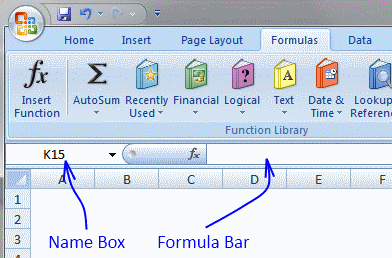Author: Oscar Cronquist Article last updated on July 29, 2017

I have written a few posts about two related tables and today I am going to show you how to work with three related tables:

### Lookups in three related tables and return multiple values

The picture below is a gif animation. It shows you how the array formula works.

The array formula looks for the Item "Router" in Item column in the first table (column B and C). The corresponding Category is B.

In table two (column E and F) there are 4 matches to Category "B". The corresponding companies in table 2 are "Gizmonic Institute", "Ewing Oil", "Sample Company" and "Mishima Zaibatsu".

In table 3 (column H and I) there are six matches to category "Company" and the adjacent Sales persons are returned in cell range C21:C27.

Array formula in cell range C21:

=IFERROR(INDEX(Table3[Sales persons], SMALL(IF(ISERROR(MATCH(Table3[Company], IF(ISERROR(MATCH(Table2[Category], IF(\$C\$19=Table1[Item], Table1[Category], ""), 0)), "", Table2[Company]), 0)), "", MATCH(ROW(Table3[Sales persons]), ROW(Table3[Sales persons]))), ROW(A1))), "")

How to create an array formula

1. Copy above array formula
2. Select cell C21
3. Click in formula bar4. Paste array formula (Ctrl + v)
5. Press and hold Ctrl+ Shift
6. Press Enter
7. Release all keys

The array formula is now surrounded by curly brackets, like this: {=array_formula}

How to copy array formula

1. Select cell C21
2. Copy cell (Ctrl + c)
3. Select cell range C22:C27
4. Paste (Ctrl + v)

### Explaining lookup array formula in cell C21

Step 1 - Check whether a condition is met and return corresponding Table1[Category] value

IF(\$C\$19=Table1[Item],Table1[Category],"")

becomes

IF("Router" = {"Drill driver"; "Angle grinder"; "Jig saw"; "Gas frame nailer"; "Table saw"; "Router"; "Mitre saw"; "Jointing machine"; "Random orbit sander"; "Planer"; "Combi drill"; "Impact wrench"; "Circlar saw"; "Rotary hammer drill"; "Biscuit joiner"}, {"A"; "B"; "C"; "D"; "A"; "B"; "C"; "D"; "A"; "B"; "C"; "D"; "A"; "B"; "C"} , "")

and returns

{"";"";"";"";"";"B";"";"";"";"";"";"";"";"";""}

Step 2 - Return the relative position of an item in an array that matches a specified value

MATCH(Table2[Category], IF(\$C\$19=Table1[Item], Table1[Category], ""), 0)

becomes

MATCH(Table2[Category], {"";"";"";"";"";"B";"";"";"";"";"";"";"";"";""}, 0)

becomes

MATCH({"A"; "B"; "C"; "A"; "D"; "B"; "A"; "C"; "D"; "B"; "C"; "C"; "B"; "A"; "D"}, {"";"";"";"";"";"B";"";"";"";"";"";"";"";"";""}, 0)

and returns

{#N/A; 6; #N/A; #N/A; #N/A; 6; #N/A; #N/A; #N/A; 6; #N/A; #N/A; 6; #N/A; #N/A}

Step 3 - Check whether a condition is met and return corresponding Table2[Company] value

IF(ISERROR(MATCH(Table2[Category], IF(\$C\$19=Table1[Item], Table1[Category], ""), 0)), "", Table2[Company])

becomes

IF(ISERROR({#N/A; 6; #N/A; #N/A; #N/A; 6; #N/A; #N/A; #N/A; 6; #N/A; #N/A; 6; #N/A; #N/A}), "", Table2[Company])

becomes

IF(ISERROR({#N/A; 6; #N/A; #N/A; #N/A; 6; #N/A; #N/A; #N/A; 6; #N/A; #N/A; 6; #N/A; #N/A}), "", {"Global Dynamics"; "Gizmonic Institute"; "Initech"; "Northern Railway"; "Sixty Second Avenue"; "Ewing Oil"; "Sonky Rubber Goods"; "Foo Bars"; "Initech"; "Sample Company"; "Gizmonic Institute"; "Energy Corporation"; "Mishima Zaibatsu"; "Monarch Co."; "Industrial Automation"})

and returns

{""; "Gizmonic Institute"; ""; ""; ""; "Ewing Oil"; ""; ""; ""; "Sample Company"; ""; ""; "Mishima Zaibatsu"; ""; ""}

Step 4 - Return the relative position of an item in an array that matches a specified value

MATCH(Table3[Company], IF(ISERROR(MATCH(Table2[Category], IF(\$C\$19=Table1[Item], Table1[Category], ""), 0)), "", Table2[Company]), 0)

becomes

MATCH({"Sample Company"; "Energy Corporation"; "Initech"; "Gizmonic Institute"; "Northern Railway"; "Sonky Rubber Goods"; "Sample Company"; "Monarch Co."; "Industrial Automation"; "Ewing Oil"; "Gizmonic Institute"; "Initech"; "Foo Bars"; "Sixty Second Avenue"; "Mishima Zaibatsu"}, {""; "Gizmonic Institute"; ""; ""; ""; "Ewing Oil"; ""; ""; ""; "Sample Company"; ""; ""; "Mishima Zaibatsu"; ""; ""}, 0)

and returns

{10; #N/A; #N/A; 2; #N/A; #N/A; 10; #N/A; #N/A; 6; 2; #N/A; #N/A; #N/A; 13}

Step 5 - Check whether a condition is met and return row number

IF(ISERROR(MATCH(Table3[Company], IF(ISERROR(MATCH(Table2[Category], IF(\$C\$19=Table1[Item], Table1[Category], ""), 0)), "", Table2[Company]), 0)), "", MATCH(ROW(Table3[Sales persons]), ROW(Table3[Sales persons])))

becomes

IF(ISERROR({10; #N/A; #N/A; 2; #N/A; #N/A; 10; #N/A; #N/A; 6; 2; #N/A; #N/A; #N/A; 13}), "", MATCH(ROW(Table3[Sales persons]), ROW(Table3[Sales persons])))

becomes

IF(ISERROR({10; #N/A; #N/A; 2; #N/A; #N/A; 10; #N/A; #N/A; 6; 2; #N/A; #N/A; #N/A; 13}), "", {1; 2; 3; 4; 5; 6; 7; 8; 9; 10; 11; 12; 13; 14; 15})

and returns

{1;"";"";4;"";"";7;"";"";10;11;"";"";"";15}

Step 6 - Return the k-th smallest row number

SMALL(array, k)

SMALL(IF(ISERROR(MATCH(Table3[Company], IF(ISERROR(MATCH(Table2[Category], IF(\$C\$19=Table1[Item], Table1[Category], ""), 0)), "", Table2[Company]), 0)), "", MATCH(ROW(Table3[Sales persons]), ROW(Table3[Sales persons]))), ROW(A1))

becomes

SMALL({1;"";"";4;"";"";7;"";"";10;11;"";"";"";15}, ROW(A1))

and returns 1

Step 7 - Return a reference of the cell at the intersection of a particular row and column

INDEX(Table3[Sales persons], SMALL(IF(ISERROR(MATCH(Table3[Company], IF(ISERROR(MATCH(Table2[Category], IF(\$C\$19=Table1[Item], Table1[Category], ""), 0)), "", Table2[Company]), 0)), "", MATCH(ROW(Table3[Sales persons]), ROW(Table3[Sales persons]))), ROW(A1)))

becomes

INDEX(Table3[Sales persons], 1)

becomes

INDEX({"Simeon Fernandes"; "Corydon Keck"; "Hija Hanna"; "Dana Leak"; "Triston Rigsby"; "Cyril Strong"; "Cyril Strong"; "Corydon Keck"; "Hija Hanna"; "Kason Leonard"; "Lewis Moya"; "Kason Leonard"; "Lewis Moya"; "Jaden Bonds"; "Doloris Hawley"}, 1)

and returns "Simeon Fernandes" in cell C21.

### Filter unique distinct values from three related tables

There are two records of Salesperson "Simeon Fernandes" and Company "Sample Company" in the last table. Filtered Salespersons in cell range C21:C25 return Simeon Fernandes only once.

Array formula in cell range C21:

=IFERROR(INDEX(Table6[Sales persons],S MALL(IF((ISERROR(MATCH(Table6[Company], IF(ISERROR(MATCH(Table5[Category], IF(\$C\$19=Table4[Item],Table4[Category], ""),0)), "", Table5[Company]), 0)))+COUNTIF(\$C\$20:C20,Table6[Sales persons]), "", MATCH(ROW(Table6[Sales persons]), ROW(Table6[Sales persons]))), 1)), "")

How to create an array formula

### Filter duplicates from three related tables

Array formula in cell C21:

=IFERROR(INDEX(Table9[Sales persons], SMALL(IF((ISERROR(MATCH(Table9[Company], IF(ISERROR(MATCH(Table8[Category], IF(\$C\$19=Table7[Item],Table7[Category], ""), 0)), "", Table8[Company]), 0)))+(COUNTIFS(Table9[Company], Table9[Company], Table9[ Sales persons], Table9[Sales persons])<=1), "", MATCH(ROW(Table9[Sales persons]), ROW(Table9[Sales persons]))), ROW(A1))), "")

How to create an array formula

### Sum values from three related tables

Array formula in cell C21:

=SUM(IF(ISERROR(MATCH(Table12[Company], IF(ISERROR(MATCH(Table11[Category], IF(\$C\$19=Table10[Item], Table10[Category], ""), 0)), "", Table11[Company]), 0)), "", Table12[Amount]))

How to create an array formula

How to copy array formula